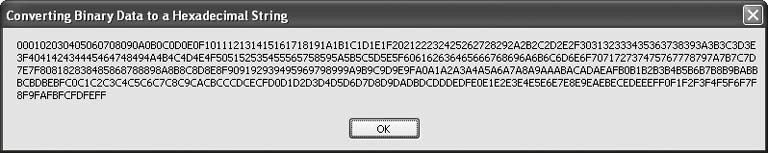# Recipe 5.5. Converting Binary Data to a Hexadecimal String

#### Problem

You need to convert a byte array to a hexadecimal string. This is handy for the display or documentation of binary data.

#### Solution

Use a bit converter to get the hexadecimal representation of each byte within a block of data. The following code generates the hexadecimal string from source data:

` Dim result As String = Replace(BitConverter.ToString( _    origBytes), "-", "") `

#### Discussion

There are several approaches to solving this problem. A quick review of some of these approaches will demonstrate several different programming techniques available to you in Visual Basic 2005.

The code samples in this recipe assume a byte array named origBytes built using the following code, which creates a byte array of length 256 containing one each of the byte values 0 through 255:

` Dim origBytes(255) As Byte For counter As Byte = 0 To 255    origBytes(counter) = counter Next counter `

The first approach is somewhat "brute force" in nature. Each byte of the array is converted to a two-character string using one of the many formatting options of the byte's ToString() method. These short strings are concatenated to the result string one at a time:

` Dim result As String = "" For counter As Byte = 0 To 255    result &= origBytes(counter).ToString("X2") Next counter `

This is fine for small arrays of bytes, but the string concatenation quickly becomes problematic as the byte count increases. The next approach uses a StringBuilder to make the concatenation more efficient for large data sources:

` Dim workText As New System.Text.StringBuilder(600) For counter = 0 To 255    workText.Append(origBytes(counter).ToString("X2")) Next counter Dim result As String = workText.ToString() `

This solution runs faster, but it seems to lack the elegance and power we expect of Visual Basic. Fortunately, the .NET Framework is full of surprises, and of useful objects too. The BitConverter object provides a shared method that converts an entire array of bytes to a hexadecimal string in one call. The resulting string has dashes between each pair of hexadecimal characters. This can be nice in some circumstances, but in this case, we're trying to create a compact hexadecimal string comprised of only two characters for each byte. The following two lines of code show how to call the BitConverter.ToString() method, and then squeeze out all the dashes using a single call to the Replace() function:

` Dim result As String result = BitConverter.ToString(origBytes) '00-3F-F7 etc. result = Replace(result, "-", "")     '003FF7 etc. `

The solution presented first in this recipe is the result of combining these two function calls into a single line of code. Figure 5-4 shows the resulting hexadecimal string displaying all possible byte values.

##### Figure 5-4. The hexadecimal string equivalent of a byte array comprised of the values 0 to 255Recipes 5.16 and 5.26 show other useful ways of modifying portions of strings.Visual Basic 2005 Cookbook: Solutions for VB 2005 Programmers (Cookbooks (OReilly))
ISBN: 0596101775
EAN: 2147483647
Year: 2006
Pages: 400